# ISEE Middle Level Math : Fractions

## Example Questions

### Example Question #31 : Fractions

Express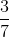as a decimal.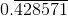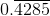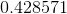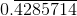Explanation:

Divide 3 by 7 to get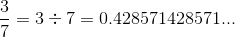or.

### Example Question #31 : How To Find The Decimal Equivalent Of A Fraction

What is the equivalent of 34%?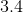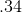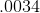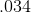Explanation:

Divide: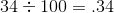### Example Question #32 : How To Find The Decimal Equivalent Of A Fraction

What is the equivalent of 68%?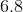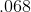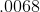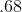Explanation:

Divide: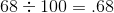### Example Question #33 : How To Find The Decimal Equivalent Of A Fraction

What is the equivalent of 29%?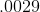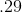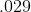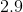Explanation:

Divide: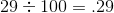### Example Question #34 : Fractions

Express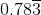as a fraction in lowest terms.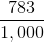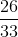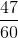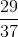Explanation:

Let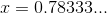Then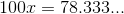and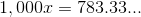Subtract: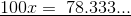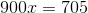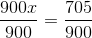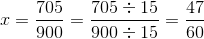### Example Question #184 : Numbers And Operations

What is the equivalent of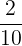?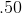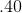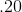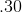Explanation:

Divide: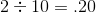### Example Question #185 : Numbers And Operations

What is the equivalent of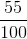?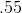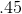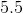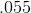Explanation:

Divide: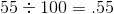### Example Question #1071 : Isee Middle Level (Grades 7 8) Mathematics Achievement

What is the equivalent of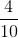?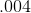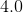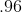Explanation:

Divide: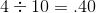### Example Question #39 : Fractions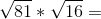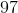Explanation:
Find the square roots: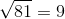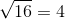Solve the equation: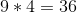### Example Question #40 : Fractions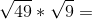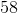Explanation:
Find the square root: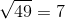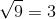Solve the equation: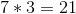### All ISEE Middle Level Math Resources